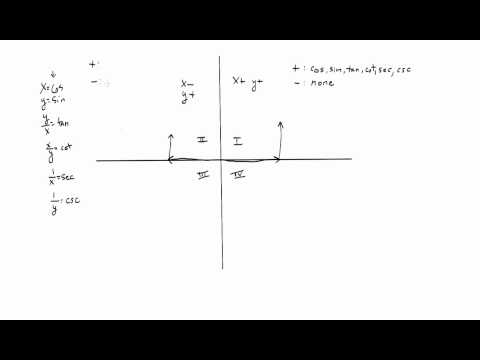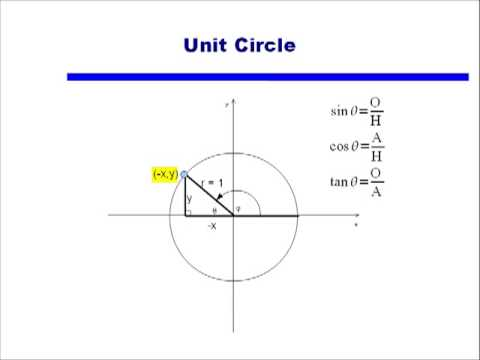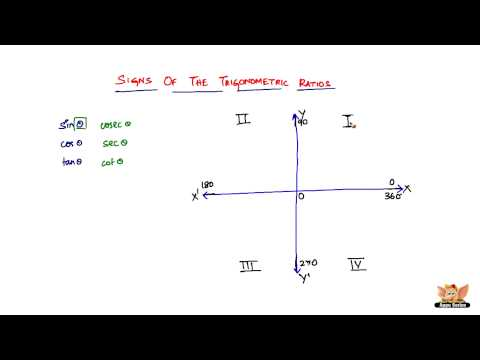## Signs of Trigonometric Ratios

Subject: Optional Mathematics

#### Overview

Any allied angle can be in the form (n × 90° ± $\theta$) where n is an integer. We can change the trigonometric ratios of the angle (n × 90° ± $\theta$) into the trigonometric ratio of an angle $\theta$.

##### Signs of Trigonometric RatiosTrigonometric Ratios of any angle

Any allied angle can be in the form (n × 90° ± $\theta$) where n is an integer. We can change the trigonometric ratios of the angle (n × 90° ± $\theta$) into the trigonometric ratio of an angle $\theta$.

1. If n is even, there will be no change in the trigonometric ratios.
i.e. sin (n × 90° ± $\theta$) ⇒ sin $\theta$
cos (n × 90° ± $\theta$) ⇒ cos $\theta$, etc.

2. If n is odd, then the trigonometric ratios change as follows:
sin (n × 90° ± $\theta$) ⇒ cos $\theta$
cos (n × 90° ± $\theta$) ⇒ sin $\theta$
tan (n × 90° ± $\theta$) ⇒ cot $\theta$
cosec (n × 90° ± $\theta$) ⇒sec $\theta$
sec (n × 90° ± $\theta$) ⇒ cosec $\theta$
cot (n × 90° ± $\theta$) ⇒ tan $\theta$

3. The sign of the trigonometric ratio of the angle (n × 90° ± $\theta$) is determined by taking into consideration that in which quadrant that angle (n × 90° ± $\theta$) lies.

Ratios of 120°

sin 120° = sin (2 × 90° - 60°) = sin 60° = $\frac{\sqrt{3}}{2}$

cos 120° = cos (1 × 90° +30°) = -sin 30° = - $\frac{1}{2}$

tan 120° = tan (2 × 90° - 60°) = -tan 60° = - $\sqrt{3}$

Ratios of 135°

sin 135° = sin (1 × 90° + 45°) = cos 45 = $\frac{1}{\sqrt{2}}$

cos 135° = cos (2 × 90° - 45°) = -cos 45 = -$\frac{1}{\sqrt{2}}$

tan 135° = tan (1 × 90° + 45°) = -cot 45 = -1

Ratios of 150°

sin 150° = sin (2 × 90° - 30°) = sin 30° = $\frac{1}{2}$

cos 150° = cos (1 × 90° +60°) = -sin 60° = -$\frac{\sqrt{3}}{2}$

tan 150° = tan (2 × 90° - 30°) = -tan 30° = -$\frac{1}{\sqrt{3}}$

##### Things to remember
1. If n is even, there will be no change in the trigonometric ratios.
i.e. sin(n × 90° ± $\theta$)⇒ sin $\theta$
cos(n × 90° ± $\theta$)⇒ cos $\theta$, etc.
2. If n is odd, then the trigonometric ratios change as follows:
sin(n × 90° ± $\theta$)⇒ cos $\theta$
cos(n × 90° ± $\theta$)⇒ sin $\theta$
tan(n × 90° ± $\theta$)⇒ cot $\theta$
cosec(n × 90° ± $\theta$)⇒sec $\theta$
sec(n × 90° ± $\theta$)⇒ cosec $\theta$
cot(n × 90° ± $\theta$)⇒ tan $\theta$
3. The sign of the trigonometric ratio of the angle(n × 90° ± $\theta$) is determined by taking into consideration that in which quadrant that angle(n × 90° ± $\theta$) lies.
• It includes every relationship which established among the people.
• There can be more than one community in a society. Community smaller than society.
• It is a network of social relationships which cannot see or touched.
• common interests and common objectives are not necessary for society.
##### Videos for Signs of Trigonometric Ratios##### Sign of Trigonometric Functions by Quadrant##### Signs of trig functions in 4 quadrants##### Questions and Answers

Soln

= sin(4×90°-45°)

= -sin45°

= -$\frac{1}{\sqrt(2)}$

Soln

= cos 1470° ($\therefore$ (-$\theta$) = cos$\theta$)

= cos(16 x 90° + 30°)

= cos 30°

= $\frac{\sqrt(3)}{2}$

Soln

= -tan 570° (tan(-$\theta$ = -tan $\theta$)

= -tan(7×90° - 60°)

= {-cot60°}

= cot 60° = $\frac{1}{\sqrt(3)}$